# Practical Exercise 8: PARAMETERS OF PLANET TRAJECTORIES

In aable 1, for each planet there is given its synodic orbital period as measured by a terrestrial astronomer. Complete the table with the calculated value of the sidereal orbital periods of the planets and derive the main half-axes of the planets.

Table 1 Synodic orbital periods of the planets and other parameters

 Planet Synodic period [day] Synodic period [year] Sidereal period [year] Main half-axis [au] Mercury 116 Venus 584 Mars 780 Jupiter 399 Saturn 378 Uranus 370 Neptune 367

ahe planets move around the Sun in the same direction, the mutual angular velocity of the Earth and the planets is calculated as the difference of their angular velocities: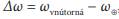, resp. v, resp.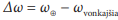. From the definition of angular velocity we get: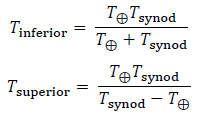We calculate the main half-axis from Kepler’s third law: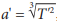, where, where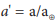, where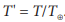.